# Excel formulas not working, not updating, not calculating: fixes & solutions

This tutorial explains the most common mistakes when making formulas in Excel, and how to fix a formula that is not calculating or not updating automatically.

Can someone imagine using Microsoft Excel without formulas? I believe no one can. And hardly anything could compare to the frustration caused by Excel formulas stop working all of a sudden. When this happens, a bunch of questions immediately flash across your mind. Why is my Excel formula not calculating? Why doesn't this formula update its value automatically? Is my Excel corrupt or is this due to some malicious virus? And how do I get my Excel to calculate and update formulas automatically again? Don't worry, most likely your Excel is all right, and you will get all the answers in a moment.

## Excel formulas not working

Symptoms: Excel formula not working correctly, it returns an error or a wrong result.

This section provides a summary of the most common mistakes people make when creating formulas in Excel and solutions to fix them.

#### 1. Match all opening and closing parentheses in a formula

As you know, the arguments of Excel functions are entered within the parentheses. In complex formulas, you may need to enter more than one set of parentheses, one within another, to indicate the order in which the calculations should take place. When creating such a formula, be sure to pair the parentheses properly so that you always have a right parenthesis for every left parenthesis in your formula.

Microsoft Excel displays the parentheses pairs in different colors as you enter them in a formula. If your formula is short of one or more parentheses, Excel displays an error message and suggests a correction to balance the pairs. Please see How to highlight and match parenthesis pairs for more information.

#### 2. Enter all required arguments in an Excel function

All Excel functions have one or more required arguments. Some functions also have optional arguments, which are enclosed in [square brackets] in the formula's syntax.

A formula must contain all of the required arguments, otherwise Excel displays "You've entered too few arguments for this function" alert.

If you have entered more arguments than allowed by the formula's syntax, you will get "You've entered too many arguments for this function" error message.

#### 3. Do not nest more than 64 functions in a formula

When nesting two or more Excel functions into each other, e.g. creating a nested IF formula, remember about the following limitations:

• In Excel 2016, Excel 2013, Excel 2010 and Excel 2007, you can use up to 64 nested functions.
• In Excel 2003 and lower, only up to 7 nested functions can be used.

#### 4. Don't enclose numbers in double quotes

In Excel formulas, any value enclosed in double quotes is interpreted as a text string.

What it means is that if you enter a formula like `=IF(A1>0, "1")`, Excel will treat number 1 as text, and therefore you won't be able to use the returned 1's in other calculations. To fix this, just remove the double quotes around "1": `=IF(A1>0, 1)`.

So, whenever you are writing a formula for numerical values, follow this simple rule: don't enclose numbers in double quotes unless you want them to be treated as text.

#### 5. Enter numbers without any formatting

When using a number in an Excel formula, don't add any decimal separator or currency sign like \$ or €.

Remember that in Excel formulas, a comma is typically used to separate a function's arguments, and the dollar sign makes an absolute cell reference.

For instance, instead of entering \$50,000 in your formula, input simply 50000, and use the Format Cells dialog (Ctrl + 1) to format the output to your liking.

#### 6. Make sure numbers are not formatted as text values

Numbers formatted as text values are another common reason for Excel formulas not working. At first sight, they look like normal numbers, but Microsoft Excel perceives them as text strings and leaves out of calculations.

The visual indicators of text-numbers are as follows:

• Numbers formatted as text are left-aligned by default, while normal numbers are right-aligned in cells.
• The Number Format box on the Home tab in the Number group displays the Text format.
• When several cells with text numbers are selected on the sheet, the Status Bar only shows Count, while usually it shows Average, Count and SUM for numbers.
• There may be a leading apostrophe visible in the formula bar, or green triangles appear in the top-left corner of the cells.

The below screenshot shows that even a simple Excel SUM formula may not work because of numbers formatted as text: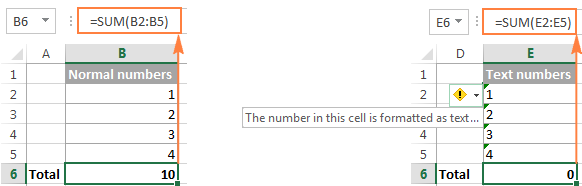To fix this, select all problematic cells, click the warning sign, and then click Convert to Number: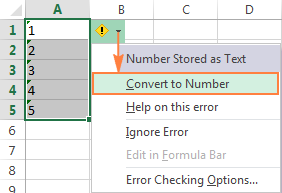In some cases, however, neither green triangles nor the warning sign appear in cells. For example, if you enclose numeric values in double quotes in your formulas, Excel assumes you want to output a text string rather than a number.

At first sight, the following formula appears to be working fine:
`=IF(A1="Good", "1", "0")`

But the problem is the returned 1's and 0's are text values, not numbers! And if you reference any cells with the above formula in other formulas, those cells won't be included in calculations. As soon as you remove "" surrounding 1 and 0 in the above formula, Excel will treat the outputs as numbers and they will be calculated correctly.

If the small green triangles do not appear in cells for some other reason, look at the Number Format box on the Home tab in the Number group. If it displays Text, try clearing all formatting for the problematic cells, and set the cells' format to Number or General. If that doesn't work, you might have to create a new column, manually input the data (e.g. copy your text-numbers to Notepad, and then back to a new column), and delete the broken column.

Another possible solution is to multiply the values in the problematic column by 1 using a simple formula like `=A1*1`. And then, copy the formula cells and paste them as values in the same or in any other column via Paste Special > Values.

#### 7. Separate function arguments with a proper character

Most of us are used to separating function arguments with commas. However, this does not work for everyone's Excel. The character you use to separate arguments depends on the List Separator set in your Regional Settings.

Comma is the default list separator in North America and some other countries. In European countries, comma is used as the decimal symbol and the list separator is usually set to semicolon.

For example, in North America you would write `=IF(A1>0, "OK", "Not OK")`, while European users of Excel should put the same formula as `=IF(A1>0; "OK"; "Not OK")`.

So, if your Excel formulas are not working because of "We found a problem with this formula..." error, go to your Regional Settings (Control Panel > Region and Language > Additional Settings) and check what character is set as List Separator there. And then, use exactly that character to separate arguments in your Excel formulas.

#### 8. Enclose workbook and worksheet names in single quotes

When referring to other worksheets or workbooks that have spaces or non-alphabetical characters in their names, enclose the names in 'single quotation marks'. For example,

Reference to another sheet:
`=SUM('Jan Sales'!B2:B10)`

Reference to another workbook:
`=SUM('[2015 Sales.xlsx]Jan sales'!B2:B10)`

#### 9. Include the full path to a closed workbook

If you are writing a formula that references a closed Excel workbook, your external reference must include the workbook name and entire path to the workbook. For example:

`=SUM('D:\Reports\[Sales.xlsx]Jan'!B2:B10)`

If the above tips do not help, try to evaluate and debug each part of your formula individually by using the F9 key and other debugging techniques explained in the following tutorial: How to evaluate and debug formulas in Excel.

## Excel formulas not updating

Symptoms: The value returned by your Excel formula does not update automatically, i.e. the formula continues to show the old value even after you've changed the values of the dependent cells.

When Excel formulas are not updating automatically, most likely it's because the Calculation setting has been changed to Manual instead of Automatic. To fix this, just set the Calculation option to Automatic again.

On the Excel ribbon, go to the Formulas tab > Calculation group, click the Calculation Options button, and select Automatic: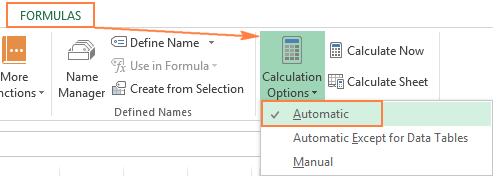Alternatively, you can change this setting in Excel Options:

• In Excel 2003, click Tools > Options > Calculation > Calculation > Automatic.
• In Excel 2007, click Office button > Excel options > Formulas > Workbook Calculation > Automatic.
• In Excel 2010, Excel 2013, and Excel 2016, go to File > Options > Formulas > Calculation options section, and select Automatic under Workbook Calculation.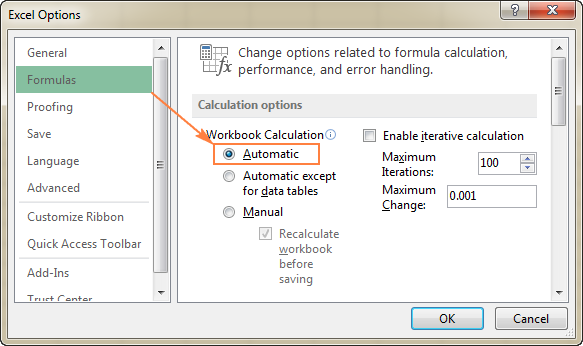### How to force Excel formulas to recalculate

If for some reason, you need to have the Calculation option set to Manual, you can force the formulas to recalculate by clicking the Calculate button on the ribbon or by using one of the following shortcuts:

To recalculate the entire workbook:

• Press F9, or
• Click the Calculate Now button on the Formulas tab > Calculation group.

To recalculate an active sheet:

• Press Shift + F9, or
• Click Calculate Sheet on the Formulas tab > Calculation group.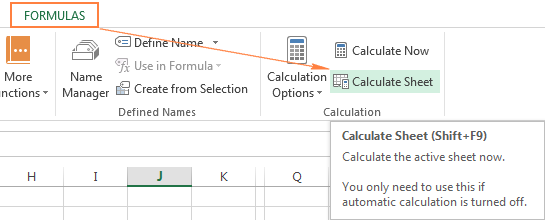To recalculate all sheets in all open workbooks, press Ctrl + Alt + F9.

If you need to recalculate only one formula on a sheet, select the formula cell, enter the editing mode either by pressing F2 or double clicking the cell, and then press the Enter key.

## Excel formulas not calculating

Symptoms: A cell displays the formula, not the result.

If your Excel formula is not working because a cell displays the function instead of the calculated value, it's because one of the following reasons.

#### 1. Show Formulas mode is turned on

The most common reason for an Excel formula not calculating is that you have inadvertently activated the Show Formulas mode in a worksheet.

To get the formula to display the calculated result, just turn off the Show Formulas mode by doing one of the following:

• Pressing the Ctrl + ` shortcut, or
• Clicking the Show Formulas button on the Formulas tab > Formula Auditing group.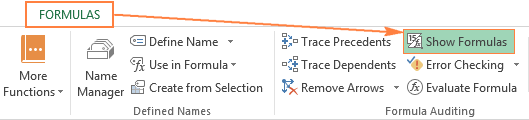#### 2. A formula is entered as text

Another frequent reason for your Excel formula not calculating is that the formula has been formatted as text. To check this, select the formula cell, and look at the Number Format box in the Number group on the Home tab: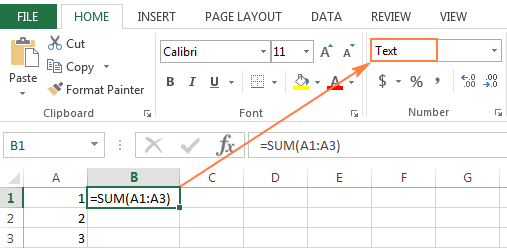If it is the case, change the cell format to General, and while in the cell press F2 and Enter for the formula to recalculate and display the calculated value.

#### 3. A formula cell has a leading space or apostrophe before the equal sign

If you have inadvertently entered a space or apostrophe (') before the equal sign, Excel treats the cell contents as text, and consequently does not evaluate any formula within that cell (a leading space often appears when you copy a formula from the web). To fix this, just remove the leading space or single quote.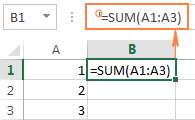This is how you deal with formulas not working in Excel. If you know any other solutions to fix formulas not updating or not calculating, please do share in comments. I thank you for reading and hope to see you on our blog next week.

## You may also be interested in

1. Hello

table format in any column locked but full table Locked and formula not automatically past nest cell

2. We had a custom =@Maxdd formula and with my recent windows 11 upgrade this stopped working, we do financial sheets and require the maxdd for 1 year period over whole column of data, previous formula was: =@maxdd(K647:K1733,1,COUNT(K647:K1733)) and now getting #name? error on the =@maxdd,

any suggestions?

3. So I'm having trouble with just trying to CONCATENATE on two cells (First Name, Last Name) to get Full Name. Before I do I test to make sure that the cell will copy/formulate, so when I set the cell =B5, it just shows =B5 as the result not as First Name. The file I'm working with was originally created in Mac Numbers and exported as Microsoft Excel. I removed the R1C1 reference style as it showed both columns and rows as numbers and not numbered rows and lettered columns. My question is, even after looking over the article, I'm still not able to get my functions to work. Any ideas?

4. COUNTIF(C10:AG10,AH8), Same formula doesnt apply in next cell, it takes from (d10:ag10,ah9) and this continues with e,f,g further.

5. My function RENDEMENT does not give the correct answer; when I enter the data of the example given in the online description of the formula I do not get the correct result 6.5% but the obviously wrong answer 4.26%. Excel does not indicate any syntax errors. this is what I entered:
RENDEMENT(DATUM(2008;2;15);DATUM(2016;11;15);5,75;95,04287;100;2;0)

6. I recently upgraded from Windows 10 to Windows 11, and along with it my Excel 2019 has switched over to Office 365 Excel. I am using the desktop app.

Without changing a single formula in one of my large workbooks, I am noticing that formula cells are not automatically updating with changes in dependent cells. I for instance have a simple column sum [SUM(X3:X54)], and it will not update with changes to the cells. I have to select the formula cell, press F2 (or click on the formula field), and press enter and then it will work.

I have verified that Calculation Options is set to Automatic. The formula cell isn't showing the formula or anything to suggest that it has been reformatted as text. It shows a correctly formatted number, but doesn't update the sum when the dependent cells are changed. Selecting the formula cell, pressing F2, and pressing Enter do not fix the problem, just force the cell to update: i.e., if I then change another dependent cell, I have to do the same thing again to get the cell to update. I presume using the Calculate Now or Calculate Sheet functions in Excel will achieve the same result.

Confoundingly, it does not affect all formula cells, and it also seems to be a temporary but recurring problem. For instance, as I tested it yesterday and today, I was able to confirm that forcing an update of the formula does not _fix_ that cell, but then just now, I entered a value into one of the dependent cells for the same formula I've been having troubles with, and the formula cell updated immediately without issue. I saved the file, closed Excel, reopened it, and the cell is updated automatically as it should. So it's almost like there is a delay.

Again, I didn't adjust any formulas, so this isn't a problem with a formula. This problem appeared only with the upgrade to Windows 11 and the change to Excel 365. I can reinstall Excel 2019 if necessary, but it seems like that should be unnecessary. Formulas not working in Excel is like a coffee shop being out of coffee.

In case it's relevant, this workbook has no links to other workbooks.

I live very rurally and only have access to really terrible internet -- 5 mbps down and 1 mbps up. (I know, I know - but they've buried the cables for fiber now and I'm getting hooked up next week). Does the Office 365 Excel on desktop offload formula calculation to the cloud? Could latency explain the problem? That at least would explain why it works sometimes and not others. I guess if no one has any other ideas, I could wait to see if the problem resolves itself when I'm hooked up to fiber next week. Otherwise, I'm at a loss.

• I have the exact same problem. Did you figure out a fix?

• Same here. All settings of Excel at automatic, no text references or show formulas and still the sheet does not recalculate. Need to click it manually everytime. If I forget it I'm working with old data... Very annoying.

• Hi, I am having the same issue. Did anyone find a fix?
Much like OP, it's simple formulas like Sums linking to other sheets within the book. No circular referencing, just straightforward calculations.

• Same, it's ridiculous you should have to hit a button for it to calculate, when it used to do it auto. All formulas are working, no circular reference, no text data fields, all worked fine prior to the update. as far as i can tell I'm signed on to microsoft, which seams could be a problem with license issues. Has anyone figured out a solution?

• Are you using a spill in your formula? e.g. "\$A1#". I was googling as I had the same problem. I took the spill out and changed it IF "" then "", and it seems to work now. I have a feeling the spill range it was dependent on was calculating slower than the cell, so it was updating on old data. putting the formula in every cell forces it to update if there's a change in the dependent column

8. Can anyone help me with this formula?

=IF(H214,1,IF(H2>16,2,IF(H2>18,3,IF(H2>20,4,IF(H2>22,5,IF(H2>24,6)))))))

For reference, anything under 13 is not important. I am trying to have it automatically define two-hour blocks with "1" for 2 hours "2" for 4 hours, etc. I hope this makes sense. Thanks

• Hi! If I understand your task correctly, the following formula should work for you:

=IF(H2>24,6,IF(H2>22,5,IF(H2>20,4,IF(H2>18,3,IF(H2>16,2,IF(H2>14,1))))))

If you have a lot of conditions, use the IFS function instead of multiple IF:

=IFS(H2>24,6,H2>22,5,H2>20,4,H2>18,3,H2>16,2,H2>14,1)

9. Hi. I have a table with some formula in columns. When i update table from last row and table spread down one row, some columns formula dont spread down! What shoul i do ?

• Hi! Unfortunately, I don't know about which formulas you are talking about. It is also important whether you are talking about an Excel table or just a table with data. Perhaps you will find useful information here: Excel - autofill formulas.

10. Thanks for the help. Here's another cause of erratic calculation in Excel. I found today that a summary total (totaling several calculated sub-totals) was erratic -- sometimes displaying zero, sometimes displaying totals that were way off -- when there was a circular reference error somewhere in the data table that contributes to the sub-totals and thus the total. I had been re-ordering entries and inadvertently created the circular reference error. Once I corrected it, the grand total calculation worked fine.

11. I am changing a formula location reference by one column, from say AG14 to AF14 and it simply then shows the formula, not the result. When changed back to original AG14, it is fine. Calc is set to Auto. The new cell AF14 is a number, not text, which I tested by performing other calcs on it with no issues.
The formula which does not work now is: =IF(Z14AI14,"HIGH","OK"))

• Sorry, for some reason the formula I copied and sent shows up incorrectly in the comment.
the formula is: =IF(Z14AI14,"HIGH","OK"))

• Same problem so let me type it out.
=IF(z14ai14,"HIGH","OK"))

• OK, I give up. Why will it not show the formula, whether I copy it or type it?!

• Hi! I assume that your formula =IF(z14 > ai14, "HIGH", "OK")) does not work because it has an extra parenthesis at the end.

• Thanks and no, it has two sets of parens. Let me try breaking the formula into pieces to see if it comes through properly.
=if(z13ai13,
"HIGH,"OK"))
The formula as stated works. When I try changing the AG to AF, the cell simply shows the whole formula as text.

• Even breaking it into pieces didn't help show it properly.
=IF(Z14 AI14, "HIGH" , "OK"))

• Still not coming through properly.
Let me describe it.
It is a nested two IF test.
If z13 is less than ag13, then return LOW, otherwise
If z13 is greater than ai13, then return HIGH
otherwise return OK
When I change the ag ref to af, the formula stops calculating and shows the text. As soon as I change it back to ag it works again.

• Hi! Unfortunately, I have not been able to reproduce your problem. This formula works correctly for me

=IF(Z13 < AF13,"Low",IF(Z13 > AI13,"High","ok"))

However, the reason may be the text format of the cell with the formula.

12. We have a set of warehouses and each one have coordinates. We would like to find distance of each warehouse from other and subsequently minimum distance warehouse by inserting {=INDEX(\$P\$1:\$CM\$1,0,MATCH(MIN(IF(\$P4:\$CM4>0,\$P4:\$CM4)),\$P4:\$CM4,0))} .This will eliminate zero distance warehouse i.e., same warehouse. But the problem is out of 76 rows ,it works fine for 68 rows whereas the formula does not work properly for 7 to 8 rows.I can't resolve the issue.Please Help.

• Hi! I can't check your formula because I don't have your data.

13. I need to copy/reproduce the Month from one worksheet to another worksheet and i use it like ='worksheet_name'!Cell# instead of showing the value of that cell its showing the same ='worksheet_name'!Cell# notation. what could be wrong?

14. Question - I have an @sum that will not add up correctly. I am trying to add up the sum of a formula =H4/60. That formula gives me the correct number. When I add a series of numbers from that formula it does not add up correctly. I am adding 872.67 & 841.67 and it gives me a total of 1714.33. It should be 1714.34. All of my settings appear to be correct....what do I have wrong? Super frustrating!

• This question has been asked many times. If your numbers are the result of formulas, then you see them in the number format that is set in the cell. This format shows the number with rounding, but the number itself does not change. Increase the digits in your number format, and you will see real numbers without rounding.
Read more: Precision of Excel calculations.

15. I enter in cell X32 the following formula =IF(AS32="Electrical Equipment",5,IF(AS32="Marine Transportation",3,IF(AS32="Oil, Gas & Consumable Fuels",8)))
In Conditional formatting, I select use formula, copy and paste cell X32's formula

I copy X32 to X29,X27 and X25. I select X29, X27 and X25 and paste formula. In Conditional formatting, I select use formula, copy and paste cell X32's formula
I want X29, X27 and X25 cells to be automatically updated when I update values in X32.

After updating X32, I select X29,X27 and X25 and try:
in Data, refresh
in Formula Calculate Now after selecting Automatic in Calculation Options
X29, X27 and X25 do not update automatically

Can you help
Thank you

16. I have values as seen below. Cell references are D61,E61,F61,G1

D E F G
131.66 131.66 131.64 131.65

If I write the formula =IF(AND(G61-F61>D61-G61,G61-F61>E61-D61),1,0) Why do I get a True value of 1? It recognises that G61-F61 > D61-G61 even though results are the same?!

• I think I realise what the issue is.

Although the values of 0.01 and 0.01 in (G61-F61>D61-G61 are identical, they are actually slightly different when put out to many more decimal places.

• Hi! If your values are calculated using other formulas, the actual values in the cells may differ from what you see by formatting up to 2 decimal digits.

17. ='Opportunity Tracking'!\$BN2*VLOOKUP('Opportunity Tracking'!\$BV2&"|"&'Opportunity Tracking'!\$BL2,CHOOSE({1,2},'FX rates'!\$I\$3:\$I\$38&"|"&'FX rates'!\$J\$3:\$J\$38,'FX rates'!\$K\$3:\$K\$38),2,FALSE)
Doesn't work says N/A

• BN is a number column, BV is a date column, BL is a text, I3:I38 is the date range and J3:J8 is the match text column like BL, K3:K38 is a number column)

• I can't check the formula that contains unique references to your workbook worksheets. Also I don't have your data.

18. Trying to create a sheet that when you select from a drop down of months - you auto populate rows/columns for day and day of week
I have this working - However I had to go in and manually update every formula in every column to get this,
I have a data column that is a date
C1=DATE(YEAR(TODAY()),MATCH(A1,"January","February","March","April","May","June","July","August","September","October","November","December"},0),1)
This pulls from A1 that is a drop down of Month -
C33 is the serial number for given date. C33 = DATEVALUE(TEXT(C1,"M/D/YYYY"))
A1/B1 is the drop down of months
I would like A2 and B2 to look like [dd][ddd] from C33 So - A2 formula is =TEXT(C33,"dd") and B2 is =TEXT(C33,"ddd")
simple enough - now to get A3 and B3 to increment one would be =TEXT(C33 + 1,"dd") this does work -
However if I try to do the corner drag to populate all the rows for said month - it increments C33 to C34 C38 .... not the 1
What am I missing here? I am sure it is obvious to the rest of you, but I can't seem to figure this out and have been mucking with this for a few hours!
Bill

19. Hi,

When completing B the static date and time will populate in F. I use this formula: =IF(B1"",IF(F1"",F1,NOW()),""). The cell format in F is Custom -> dd/mm/yyyy hh:mm.

It worked from last year until two weeks ago. Since two weeks ago, F is blank when I select anything in B.

I managed to find this formula for a different sheet: =IF(AND(SIC!J2>=0,"",SIC!J20),NOW(),""). But it is not static and I need it to be like with the first formula when it worked.

How can I fix it?

20. This helped alot! Thank you!

21. So I just corrected my issue by reading one of your other articles.

Thank you so much for making this available to us!!

22. I have an issue with my formula not updating the refence cells when dragged down the column.
The formula works fine with all calculations, however, when I drag it down it does not update the reference cells so I get the same result for the entire column.
In order to get the correct result I have to manually update the formula in each cell.

I tried using this same formula in a brand new workbook to discard formatting issues and I got the same result.

• I have the same issue but my cells do not have absolute values. When I drag whe formula down it does not update to the next line. This is the formula im using because i want to count differente cells that are not in a sequence and meet a criteria:

=SUM(COUNTIF(INDIRECT({"C22","H22","M22","R22","W22","AB22","AG22","AL22"}),"x"))

I have 146 lines, but when dragging down I need it to update to the following lines 23, 24 and so on.

23. Bless you!
Everytime I get frustrated with Excel, Ablebits has an array of solutions.
thank you for making this resource available!!

24. It's ok! I've worked it out. The data in the other excel document was not formatted to Number so I updated the format and all now works. Thank you anyway! :-)

25. Hi, I have a simple SUM formula not working (gives 0.00 results) due to the other formulas in the cells that the Sum formula is adding up from. I know this because I experimented by removing the other formulas and just manually input some numbers and the SUM formula worked!
But I want to keep the other formulas as they are getting data from another excel document eg
Col D =VLOOKUP('[Reserves Leave Allowances_24.01.23.xlsx]Allowances'!A2,'[Reserves Leave Allowances_24.01.23.xlsx]Allowances'!\$A:\$I,9,FALSE) and similar formula in Col E (these formulae work fine).
However, the SUM formula which is in Col I =SUM(D2:H2) is just resulting in 0.00
All data is in number format.
There isn't any data (at the moment) in Cols F-H.

Surely other formulas should not be affecting further formulas especially a simple one like SUM?

26. I'm trying to get ANY formula to work currently.
In cell E4 I enter a simple test: =CONCAT(CQ4;CQ5)
In cells CQ4 and 5, I have "abc" and "xyz", of type General.
On clicking enter for this formula, it just displays the formula.
Ensuring Automatic calculation on cell E4 does not work.
Forcing the calculation does not work.
Manual Calculation does not work.

Formulas do however work for cells in other columns.

There is no Protection or locking on the E column.

Other simple formulas like =sum(1;2), also do not work in the E column.

Microsoft® Excel® for Microsoft 365 MSO (Version 2208 Build 16.0.15601.20446) 64-bit

• Nevermind... I just needed to format the E column to General or similar to make the formulas work.

27. Thank you so much

28. Didn't work Automatic calculations in my worksheet.i just tried to all the methods of above mentioned but it not corrected.

• Same situation with me here, but only 1 cell has a problem. Anybody to answer, please?

29. I really appreciate you for this knowledge. You have really save my day

30. tried plotting scatter plot. some times accepts all the values of the series and sometimes only 1

31. I have formulas that work on one tab (year 2019) , and on the next years tab (2020) the formula works on 2 lines but the rest of the cells are coming back zero. All references seem to be correct since 2019 worked perfectly. They are all formatted as a number, and I can see the backup that the formula should be picking up. Any ideas on how to fix this? The formula is a SMIFS.

• Hi!

• That kind of error can happen when you copy from one sheet to another. To correct it, ensure you paste it in similar row and column as the sheet you copied from

32. is get.workbook function available in excel 2016?

33. You are my savior!!!! Thanks a lot for the information! I have been suffering so many years because it was not calculating simple data, i had to click thousands of times the F2 button to put the numbers in the right format!

• Sir, what did you do ?

34. I have a list of employee data and I have cells that are dependent on others others, I used formulas to calculate values like date of retirement, years spent in service and so on. My problem is I want to use conditional formatting on rows that empkjee is either 59 years or above or has spent 34 or more years in service. When I used =\$H2>=59 I got the desired result,but \$O2>=34,the whole sheet gets formatted. I tried combining the 2 conditions using =OR( \$O2>=34,=\$H2>=59) it still didn't work

35. A list of numbers all derived from formulae (e.g. IMPRODUCT(B3,C3) will not sum. They are not text. The only numbers included in the calculation are those not derived from a formula. What is going on?

• Hi!
The IMPRODUCT function returns numbers in text format. Please check out this article to learn how to convert text to numbers in Excel.

36. Following up on your reply to my question, cells F2 and E2 do not have any formula or circular reference, they are cells with value entered.
e.g E2 has value 122,549,069 and F2 has value 122,548,865 and formula in 'G2' column is =F2-E2. This formula is not getting refreshed automatically, we need to open the excel and click inside cell 'G2' to see the formula being applied

37. Hi,
I have a excel sheet which has a formula in one column say 'G' column, the formula(F2-E2) in the 'G' column is to substract values from two other columns. But the formula is not getting automatically applied on opening the excel, we need to click inside the 'G" column after that the formula gets applied.
I tried changing format of the column 'G' to 'Number' and also tried Calculation option to 'Automatic', still its not working.
Please suggest any other solution which can work

• Hi!
I don't know what formulas are in cells F2 and E2. Perhaps they have repeated calculations or circular references.

38. A clarification on my previous comment. I create the formulas through a macro, using the RefersToR1C1:= option.

I build the formula by putting code like this in a loop.
YTDPointsVar = YTDPointsVar & "RC" & Range("Points" & TheWeek).Column & ","

Then I build the formula with this code:
ActiveSheet.Cells(TeamRow(TheTeam), Range("YTD_Points").Column).Formula = "=sum(" & YTDPointsVar & ")"

I don't have the R1C1 reference style selected in File, Options, Formulas. In the past the above code would automatically convert to this style;
=SUM(\$I2,\$Q2,\$Y2,\$AG2,\$AO2,\$AW2,\$BE2,\$BM2,\$BU2,\$CC2,\$CK2,\$CS2,\$DA2,\$DI2,\$DQ2,\$DY2,\$EG2,\$EO2,\$EW2,\$FE2,\$FM2,\$FU2,\$GC2,\$GK2,\$GS2,\$HA2,\$HI2,\$HQ2,) and it would calculate properly. Now, using the same code, not in compatibility mode, it displays in the RC format and no longer calculates the formula.

• Hi!
Change the macro to create a link in R1C1 reference style.

39. I have used formulas of the following style in a workbook since 1994: =max(rc13,rc21,rc29)

These have always worked. I have been saving in compatibility mode until a week ago. I saved the workbook as a .xlsm file instead of a .xls file and no longer use compatibility mode. Now the formulas don't calculate. I have automatic calculate on and have selected F9, etc. When I select the "R1C1 reference style" option under "File, Options, Formulas" the formulas automatically change to something like this: R[-112]C,R[-104]C,R[-96]C463]).

In my previous versions of the workbook I did not have "R1C1 reference style" selected and the formulas still updated properly. I'm running Excel 2013 on Windows 10.

40. My IF formula has worked no problem for 38 rows of my spreadsheet (adding a sequential number) but now no longer works and I believe I have tried every troubleshoot option above. When I compare the formulae the only difference is the row number which has been the same for the formula in every other row. Please can you help?

=IF(C38="","",LEFT(C38,1)&"-"&RIGHT(YEAR(D38),2)&"-"&MONTH(D38)&"-"&WEEKNUM(D38)&"-"&RIGHT(A38,4)) - This formula works for row 38 & all previous rows and returns C-22-8-35-1036

=IF(C39="","",LEFT(C39,1)&"-"&RIGHT(YEAR(D39),2)&"-"&MONTH(D39)&"-"&WEEKNUM(D39)&"-"&RIGHT(A39,4)) - This formula works for row 39 & returns
C-22-4-17-1037

=IF(C40="","",LEFT(C40,1)&"-"&RIGHT(YEAR(D40),2)&"-"&MONTH(D40)&"-"&WEEKNUM(D40)&"-"&RIGHT(A40,4)) - This formula does not work for row 40 & returns C-22-4-17- where it should return C-22-4-17-1038.

Unique identifier
The first letter is the source from column C.
2nd two digits are the year (column D)
Next two are the month (column D)
Next two are the week (column D)
Last is (should be) the next sequential number

Thanks

• Hi!
I can't check your formula because I don't know what is written in cell A40. The RIGHT function extracts the last 4 characters from this cell. It's your sequential number.

41. When I paste new data in a sheet excel is stopping calculation if where a row is blank

42. Hi!

I have a lot of data in my excel , around 160 000 rows and I have a few formulas in separate column that are supposed to read from the data. I am manually calculating it ,and for some reason it only calculates part of it and then I have to refresh and calculate again ,it happens on smaller samples as well and I never really know how many times should I recalculate to make sure it's fully calculated. Could you please let me know if you have any idea what can be causing it?

Best Regards,
Nino

• Hello!
If you use the F9 key, then the formulas changed since the last calculation and the formulas that depend on them are recalculated.
Try CTRL+SHIFT+ALT+F9 key. All formulas in all open workbooks will be recalculated, regardless of whether they have been modified since the last recalculation.
You can read more details here: Excel calculations: automatic, manual, iterative.

43. Dear Sirs:

I have this formula
=INDICE(Chiesa[Switch]|COINCIDIR(Instalaciones!\$I10|Chiesa[IP]|0))

if I edit it, for example, adding an extra space at the end, and then removing it, it will never be calculated again. although, formating to text, general, recalculate, etc...

there is no choice for me to edit it and include an if.na , or other function.

Please, do you have any ideas?

Thank you

Alfredo

• Hello!
Please write the formula for the English version of Excel and explain the problem in more detail.

44. I have at least two cells the formulae are not working in a .xlsm file. The formulae are in the cells but the displays are blank, and are on different worksheets. In both cases the adjacent formulae, the only difference being the column/row numbers, are working as intended.

The simplest of the two formulae is =IF(MAX(Y14:Y17)>NOW()-\$B46,MAX(Y14:Y17),0)

Automatic calculation is set, format for the formula below is set to date (and number on the one below).

I had been trying to find why the following formula wasn’t working for a couple of days, when today I realised that the date formula above also wasn’t working now.

=INDEX(Nation2!G\$4:G\$9999,MATCH(INDIRECT("A"&ROW()),Nation2!B\$4:B\$9999,0))

I knew that the first formula was working yesterday. For the last three months I have been saving this file as a back-up yet, when I opened two of the back-ups, the corresponding formulae in those two cells were no longer working correctly.

On the second formula, the ‘A’ cell value date is ‘31/12/22’ [UK date], and there is one ‘31/12/22’ date in the ‘B’ range, and the corresponding ‘G’ value was not being displayed. However, if I changed the ‘A’ and ‘B’ dates to virtually any other date, the correct ‘G’ value would be displayed. This is true if the dates were any month end bar Dec 22 (including Dec 21 and Dec 23), and having seen it work with ‘15/12/22’ I changed just the ‘15’ element but to no avail.

• Hi!
Unfortunately, you didn't explain and I can't guess what doesn't work in your formula.
If in column B the desired date is in position 4, then the fourth value from column G is returned.

45. I have a workbook with many different sheets. On one sheet (Master Project List), there are 17 columns and 365 rows of information. I have multiple other sheets that go through this list of projects and separate them into the correct ones (i.e., work plans, under budget projects, over-budget projects, etc.).

The problem I am having is that if I delete a row from the Master Project List sheet, the data on those other sheets disappears (because it can't reference the cells in the rows that I deleted), and I have to manually copy and paste them back in for it to show data again. (refreshing the calculations doesn't work).

FORMULAS USED ON WORK PLANS SHEET (for example):
Column A: =IF('Master Project List'!C2="Work Plan",TRUE,FALSE)
Column B: =IFERROR(INDEX('Master Project List'!A\$2:A\$674,SMALL(IF(\$A\$2:\$A\$1160,ROW(\$A\$2:\$A\$1160)-ROW(\$A\$2)+1),ROWS(\$1:1))),"")

Is there any way to fix it so that when I delete a row on the Master Project List, the cells with TRUE/FALSE can change their reference cell to the row below it (or just delete themselves?

• Hi!
I'm really sorry, looks like this is not possible with the standard Excel options.

• I've been using this workaround:
To "delete" Row 25 from 'Master Project List'
Highlight Row 26 through the last row that you're using, PLUS the next row or two. This will ensure that the last (previously populated) row doesn't become a duplicate of the 'new' last row.
(If the last row you're using is 325, you'll highlight rows 26 thru 327)
COPY
Select cell A25
PASTE
That will keep all dependent formulas intact.

46. Date....................Day.............Time In............Time Out...........Time In............Time Out...........Total Hours
5/24/2022.........Tuesday.......08:05AM........12:00PM...........07:00PM ..........12:01 AM ...........#VALUE!

I used this =(E9-D9)+(H9-G9) Formula. But my computer didn't work. I Go to Region-Additional Date & Time- number-list operator. But didn't work formula.

47. Date Day Time In Time Out Time In Time Out Total Hours
5/24/2022 Tuesday 08:05AM 12:00PM 07:00PM 12:01 AM #VALUE!

I used this =(E9-D9)+(H9-G9) Formula. But my computer didn't work. I Go to Region-Additional Date & Time- number-list operator. But didn't work formula.

• Hi!
If I understand correctly, text is written in E9. You subtract a number (date) from the text.

48. I have a budget spreadsheet with a separate sheet for each region and a totals sheet.
I want to calculate a simple percentage of the total budget used year to date - on the sheet itself it should simply be F2/G2 = xx% - but it always shows 0% as the answer.

F2 is a sum of B2+C2+D2+E2 (and each of those values pulls from another sheet per row)
G2 is simply pulling from another cell reference

so effectively it is a formula calculating cells that are also results of formulas. (of course if I copy the data into a new excel file and the values become absolute, it is a very basic formula that works.

can this work?

• Hello!
If cells F2 and G2 contain numbers and you use the formula =F2/G2, then check the percentage format in the cell with the formula.

49. I have Office365 Excel and my formulas don't work at all. Is there a security issue I need to fix? Getting a string value from Column A in Column Z, I used =A365 shows as = [@[COL_A]] not the value from Column A. As well, using

=XLOOKUP(\$C:\$C,'ExcelSheet2.xlsm]Exceptions'!\$I:\$I,'[ExcelSheet2.xlsm]Exceptions'!\$N:\$N,"No Match",0,1)

does not convert to the expected display value but simply shows the formula itself.

• Hello!
The @ symbol means an absolute structured reference to the current row.
I can't check the formula that contains unique references to your workbook worksheets.
However, it is written not quite correctly. Try like this -

=XLOOKUP(\$C:\$C,[ExcelSheet2.xlsm]Exceptions!\$I:\$I,[ExcelSheet2.xlsm]Exceptions!\$N:\$N,"No Match",0,1)